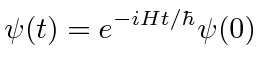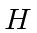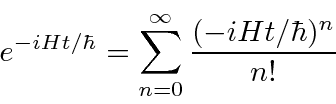## The Time Development Operator *

We can actually make an operator that does the time development of a wave function. We just make the simple exponential solution to the Schrödinger equation using operators.whereis the operator. We can expand this exponential to understand its meaning a bit.This is an infinite series containing all powers of the Hamiltonian. In some cases, it can be easily computed.is the time development operator. It takes a state from time 0 to time.

Jim Branson 2013-04-22